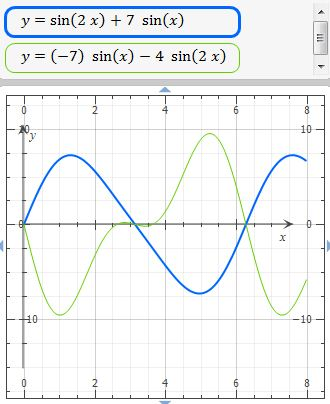# Find the points of inflection of the graph of the function. f(x) = 7 sin x + sin 2x, [0, 2\pi]...

## Question:

Find the points of inflection of the graph of the function.

f(x) = 7 sin x + sin 2x, {eq}[0, 2\pi] {/eq}

(x, y)= ( ) (smallest x-value)

(x, y)= ( ) (largest x-value)

## Inflection point

A function with one independent variable has an inflection point where its second derivative is zero, therefore an inflection point is found solving the second derivative expression, or with the second derivative graph, identifying the x-axis interceptions.

The function is:

{eq}\displaystyle \ f(x) = 7 sin x + sin 2x \\ {/eq}

The first derivative is:

{eq}\displaystyle \ f'(x)= 7\,\cos \left( x \right) +2\,\cos \left( 2\,x \right) \\ \displaystyle \ f'(x)= 7\,\cos \left( x \right) +4\, \left( \cos \left( x \right) \right) ^{2}-2 \\ \displaystyle \ f'(x)= \left( \cos \left( x \right) +2 \right) \left( 4\,\cos \left( x \right) -1 \right) \\ {/eq}

The second derivative is:

{eq}\displaystyle \ f''(x) = -7\,\sin \left( x \right) -4\,\sin \left( 2\,x \right) \\ {/eq}

Inflection point exist where {eq}\ f''(x) =0 {/eq} so,

Thun, the inflection points are:

{eq}\displaystyle 0 = \ f''(x) \; \Rightarrow \; 0= -7\,\sin \left( x \right) -4\,\sin \left( 2\,x \right) \\ {/eq}

With algebraic manipulation is impossible to find the roots or inflection points of this equation, but we can graph the second derivative and see its x-axis interceptions.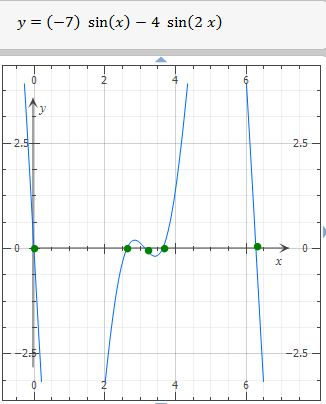With close up: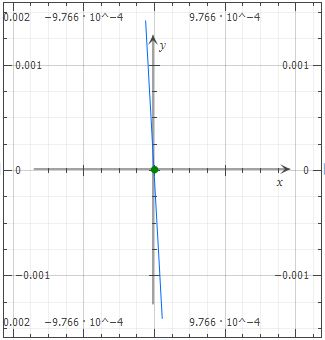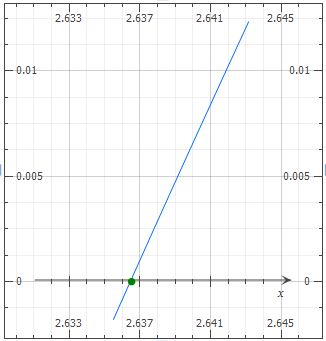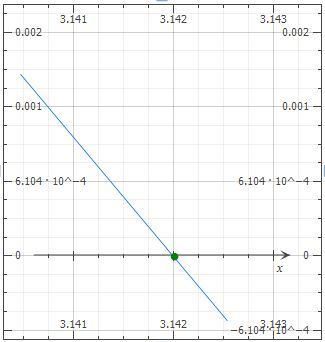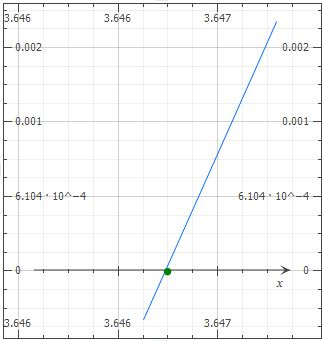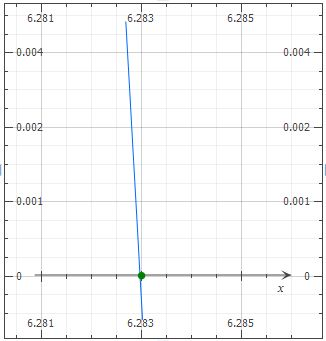Conclusion:

The inflection points are

{eq}\displaystyle (0,f(0)) \; \Rightarrow \; (0, 0) \\ \displaystyle ( 2.637,f(2.637)) \; \Rightarrow \; (2.637, 2.537758046) \\ \displaystyle ( 3.142,f(3.142)) \; \Rightarrow \; (3.142, - 0.002036732062) \\ \displaystyle ( 3.636,f(3.636)) \; \Rightarrow \; (3.636, - 2.486195334) \\ \displaystyle ( 6.283,f(6.283)) \; \Rightarrow \; (6.283, - 0.001667764601) \\ {/eq}